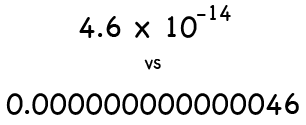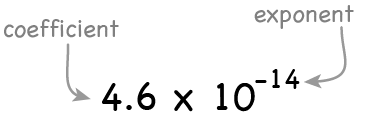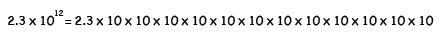# Scientific Notation

Some people have a strong resistance to using scientific notation*. Almost every time I teach an introductory science class, I have one or two students with strong math skills who insist on doing all of their calculations in standard notation. Doing this invariably results in mistakes that lead to lost points on exams and homework.

Video Overview

Making sense of scientific notation does take a bit of effort, but once learned, it becomes an effective way to save time and avoid mistakes when working with extremely large and small numbers. This is especially useful when using calculators or computers for applied work. It is much easier to avoid mistakes when writing 4.6x10-14 as compared to writing 0.000000000000046.Converting a number from standard to scientific notation involves moving the decimal place until there is one digit to the left of the decimal, then multiplying that value by ten raised to the same power as the number of places moved. 300 written in scientific notation is 3x102; spoke as "Three times ten to the two." Ten raised to the power of two indicates that to convert the value back to standard notation, the decimal needs to move two places. The sign of the exponent* depends on the direction the decimal moves.

Move the slider to compare values in standard and scientific notation

All values written in scientific notation have the same structure: The number itself, followed by a multiplication symbol, then the number 10 raised to a power.

The number is called the coefficient. The power 10 is raised to is called the exponent. The coefficient can be any real number. The exponent must be an integer, although it can be negative.A positive exponent means the number is greater than one, and the decimal moves to the right to convert from scientific to standard notation. 3.0 x102 becomes 300.

A negative exponent means the number is less than one, and the decimal moves to the left to convert from scientific to standard notation. 3.0 x10-2 becomes 0.03.

Moving the decimal the same number of places 10 is raised to works because moving the decimal one place is the same as dividing or multiplying the number by 10:

• Step 1 - divide 300 by 10: 300 ÷ 10 = 30
• Step 2 - divide the result of step 1 by 10 again: 30 x 101 ÷ 10 = 3

Since we had to divide by 10 two times to get from 300 to 3, the exponent for the scientific notation form is 2. This shows that the original number can be recreated by multiplying 3 by 10 two times.

Therefore, 3.0 x 102, 3.0 x 10 x 10 and 300 all represent the same value.

The same pattern works regardless of the size of the number. 2,300,000,000,000 can be written as 2.3 x 1012 which says it is 2.3 multiplied by 10 12 times.Values that are less than one work the same way. The difference is that converting from standard to scientific notation involves multiplying by 10, not dividing. Multiplying by 10 moves the decimal to the right. To write the number 0.0003 in scientific notation, 0.0003 is repeatedly multiplied by 10 until the first non-zero number (in this case a 3) is just to the left of the decimal place:

• Step 1 - multiply by 10: 0.0003 x 10 = 0.003
• Step 2 - multiply by 10 again: 0.003 x 10-1 x 10 = 0.03
• Step 3 - multiply by 10 again: 0.03 x 10-2 x 10 = 0.3
• Step 4 - multiply by 10 again: 0.3 x 10-3 x 10 = 3

This conversion took 4 steps so the number is multiplied by 10 raised to the negative of the number so steps indicating that to getting back to the original number requires dividing by 10 4 times. The exponent is negative because the original number is less than one.

In addition to making it easier to express large and small numbers, scientific notation makes it easier to compare values. Writing two values as:

0.000000035 and 0.0000000098

makes it difficult to see at a glance which one is bigger while writing them as

3.5 x10-8 and 9.8 x10-9

makes it much clearer that the first one is larger.

The most common way to write values in scientific notation is with the x10exponent structure. With the advent of computers and calculators, the letter E replaces "x10" so that 3200 can be written as 3200, 3.2E3, or 3.2 x103.

Try the scientific notation practice problems to test your understanding of the concepts covered by this illustration.

Related content: